### Pipe, Manning

This is a straight pipe with constant section and head losses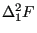defined by the Manning formula: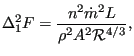(148)

where n is the Manning coefficient (unit: time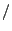length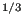),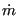is the mass flux, L is the length of the pipe,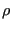is the liquid density, A is the cross section of the pipe and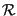is the hydraulic radius defined by the area of the cross section divided by its circumference (for a circle the hydraulic radius is one fourth of the diameter). The following constants have to be specified on the line beneath the *FLUID SECTION, TYPE=PIPE MANNING card:

• area of the cross section
• hydraulic radius of the cross section (area/perimeter)
• Manning coefficient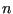The length of the pipe is determined from the coordinates of its end nodes. Typical values forare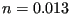smfor steel pipes and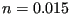smfor smooth concrete pipes (these values are for water. Notice that, since the dynamic viscosity does not show up explicitly in the Manning formula,may be a function of the viscosity).

By specifying the addition FLEXIBLE in the type label the user can create a flexible pipe. In that case the user specifies two nodes, the distance between them being the radius of the pipe. These nodes have to be genuine structural nodes and should not belong to the fluid network. The distance is calculated from the location of the nodes at the start of the calculation modified by any displacements affecting the nodes. Consequently, the use of the *COUPLED TEMPERATURE-DISPLACEMENT keyword allows for a coupling of the deformation of the pipe wall with the flow in the pipe. The following constants have to be specified on the line beneath the *FLUID SECTION, TYPE=PIPE MANNING FLEXIBLE card:

• node number 1
• node number 2
• Manning coefficientExample files: artery1, artery2, centheat1, centheat2, pipe, piperestrictor.